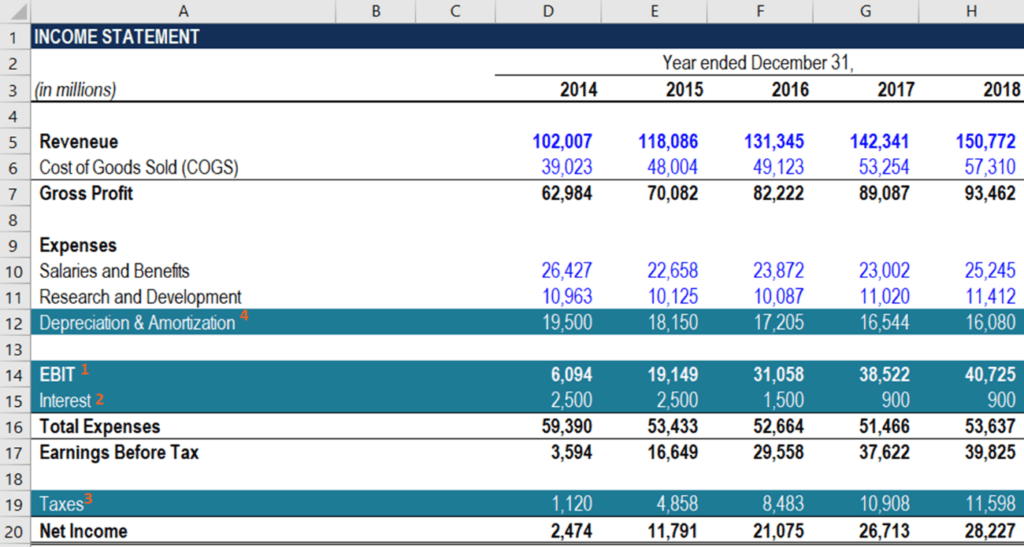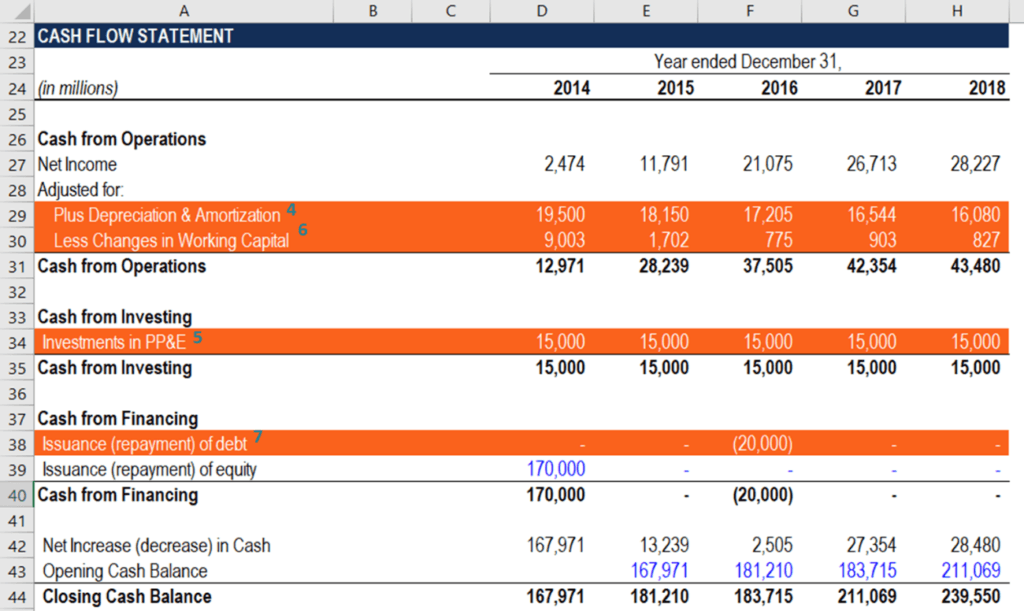# How to Calculate FCFE from EBIT?

FCFE = EBIT – Interest – Taxes + Depreciation & Amortization – ΔWorking Capital – CapEx + Net Borrowing

## How to Calculate FCFE from EBIT?

Free Cash Flow to Equity (FCFE) is the amount of cash generated by a company that can be potentially distributed to its shareholders. FCFE is a crucial metric in one of the methods in the Discounted Cash Flow (DCF) valuation model. Using the FCFE, an analyst can determine the Net Present Value (NPV) of a company’s equity, which can be subsequently used to calculate the theoretical share price of the company.

The FCFE is different from the Free Cash Flow to Firm (FCFF), which indicates the amount of cash generated to all holders of the company’s securities (both investors and lenders).### FCFE from EBIT Formula

Earnings before interest and taxes (EBIT) is one of the most crucial metrics of a company’s profitability. The measure assesses all the company’s incomes and expenses, excluding the interest and tax expenses.

As net income can be directly derived from net income, one of the methods of calculating the free cash flow to equity (FCFE) implies the use of EBIT. Recall that the company’s net income is related to EBIT through the following equation:

##### Net Income = EBIT – Interest – Taxes

Thus, we can substitute net income in the FCFE from net income formula with the equation above:

##### FCFE = EBIT – Interest – Taxes + Depreciation & Amortization – ΔWorking Capital – CapEx + Net Borrowing

Where:

• FCFE – Free Cash Flow to Equity
• EBIT – Earnings Before Interest and Taxes
• ΔWorking Capital – Change in the Working Capital
• CapEx – Capital Expenditure

The abovementioned approach to calculating the FCFE provides a more detailed overview of the composition of the free cash flows to equity (FCFE). Note that this level of granularity is not always required in a financial model.

In some cases, it can result in negative effects as it complicates the comprehension of a model. However, it is acceptable to apply this variation of FCFE calculation when the assessment of the company’s profitability from its regular business activities is essential.### FCFE from CFO Formula and Financial Statements

An analyst who calculates the free cash flows to equity in a financial model must be able to quickly navigate through financial statements. The primary reason is that all the inputs required for the calculation of the metric are taken from the financial statements. The guidance below will help you to quickly and correctly incorporate the FCFE from EBIT calculation into a financial model.

1. EBIT: The company’s earnings before interests and taxes (EBIT) are recorded on the company’s income statement.
2. Interest: The company’s interest expenses are located on the income statement after the EBIT.
3. Taxes: The tax payments can also be found on the income statement after the earnings before taxes (EBT).
4. Depreciation & Amortization: The depreciation and amortization expense is recorded on the company’s income statement under the Expenses section. The section follows the company’s gross profit. Similar to net income, the depreciation and amortization expense is also listed on the cash flow statement on the Cash from Operations section.
5. CapEx: The capital expenditure (CapEx) can be found on the cash flow statement within the Cash from Investing section.
6. Change in Working Capital (can also be denoted as ΔWorking Capital) is calculated in the company’s cash flow statement within the Cash from Operations section.
7. Net Debt: The net debt amount is also located on the cash flow statement under the Cash from Investing section.

CFI offers the Financial Modeling & Valuation Analyst (FMVA)™ certification program for those looking to take their careers to the next level. To keep learning and advancing your career, the following resources will be helpful:

• Cost of Equity
• EBIT vs EBITDA
• Operating Income
• Valuation Methods

### Financial Analyst Training

Get world-class financial training with CFI’s online certified financial analyst training program!

Gain the confidence you need to move up the ladder in a high powered corporate finance career path.

Learn financial modeling and valuation in Excel the easy way, with step-by-step training.